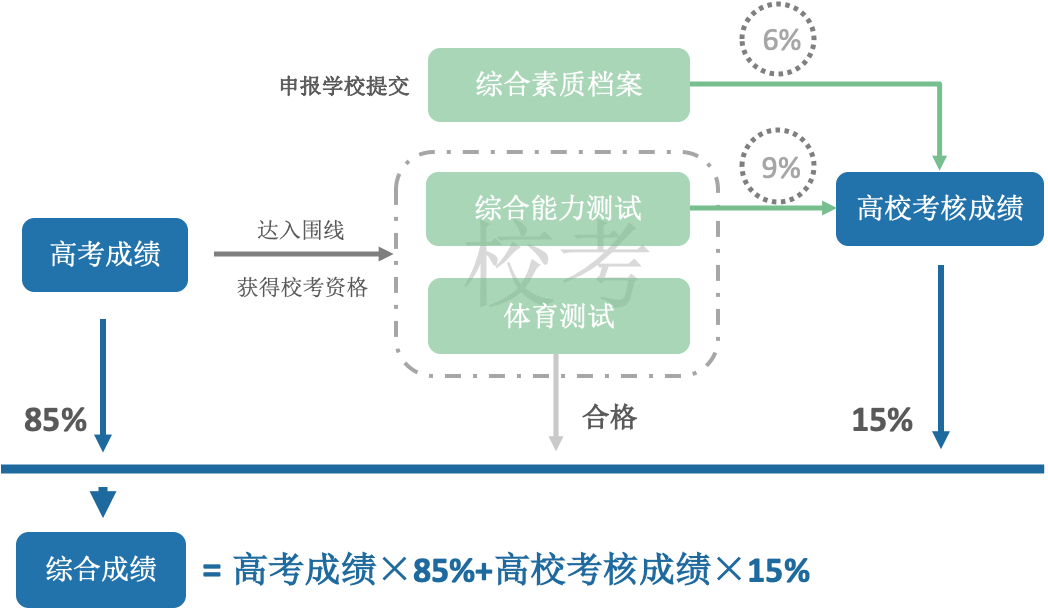0%### 二零二零年四月十九日

「现在的努力不是为了未来能够功成名就，而是为了未来有能够自己选择的权利。」

### 二零二零年四月二十二日1.小宫向他告白之前。

2.小宫向他告白之后。在明确回复小宫之前之后都没有”暧昧“的行为，明确拒绝小宫之后态度也很明确。

### 二零二零年四月二十五日### 二零二零年四月二十九日### 二零二零年五月三日

「因此，那时无聊的自信或者自尊是毫无意义的，就算未能达成，命运的安排也不会差。我相信未来一定更好，因此，只需要看清现在，在现在能选择的选项中权衡即可。 ----KSkun」

### 二零二零年五月四日

$P(A_n^1)=3\times\frac{1}{3^n}\sum_{i=1}^{n-1}\binom{n}{i}=\frac{2^n-2}{3^{n-1}}\\ P(A_n)=1-P(A_n^1)-\frac{1}{3^n}\times3=1-\frac{2^n-2}{3^{n-1}}-\frac{1}{3^{n-1}}=\frac{3^{n-1}-2^n+1}{3^{n-1}}\\ P(A_n)=P(A_{n-1})+\frac{P(A_{n-1}^1)}{3}$### 二零二零年五月六日$f_n$表示课搭载$n$个乘客的飞机上，$n$号乘客最终做到$n$号位置上的概率。则有：

$f_1=1\\f_n=\frac{1+\sum_{i=2}^{n-1}f_{n-i+1}}{n}(n\ge 2)$

$f_1=1\\f_n=\frac{1}{2}(n\ge 2)$

### 二零二零年五月九日

SJTU给的优惠是校测“最优”，直接面试和体侧。感觉还行(?)

### 二零二零年五月十日

**没必要拘泥于一次模考的成绩，总结自己的问题才是重中之重。**虽然考得好可以增长信心，考得不好也不能妄自菲薄啊！

### 二零二零年五月二十四日

Don’t forget. Always,somewhere,someone is fighting for you. As long as you remember her,you are not alone.——魔法少女小圆」

Don’t forget. Always,somewhere,someone is fighting for you. As long as you remember him,you are not alone.——ViXbob」